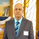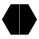# ThreeDoves Strategy Long/Short Term - synapticex

צפיות ‎1890‎
I was trying to test the GalloBias indicator so I decided to create a basic strategy for it. Maybe it is a bit naive, because there is no proper risk management and explicit costs implemented in it. So keep in mind the probably won't work on a real account.
http://synapticex.com/

Notes:
DirectionGalloLength: it is the period input for GalloBias
DirectionGalloTF: it should be greater than the current Time Frame but not too much
EntryKamaLength and ConfirmationKamaLength are the period inputs for the relative kama MAs that function as a filter for the shorter term trend.
ROClength for the Rate of Change indicator used for entry, so a greater input will lead to less trades and viceversa.

Once the GalloBias finds the general trend the bot is allowed to take trades only in that direction and when kama confirms the same as soon as the roc cross the zero line.
```//@version=2
//synapticex.com
DirectionGalloLength = input(15, minval=1)
DirectionGalloTF = input(title="DirectionGalloTF", type=resolution, defval = "D")
EntryKamaLength = input(9, minval=1)
ConfirmationKamaLength=input(4, minval=1)
ROCLength=input(7, minval=1)

gallo(length)=>
volatility = sum(abs(close-close), length)
change = abs(close-close[length-1])
er = iff(volatility != 0, change/volatility, 0)
sc = pow((er*(0.666666-0.064516))+0.064516, 2)
k = nz(k)+(sc*(hl2-nz(k)))

galloDir = security(tickerid,DirectionGalloTF, gallo(DirectionGalloLength))
galloA = sma(galloDir, 5)
kamaEn = security(tickerid,period,gallo(EntryKamaLength))
kamaConf = security(tickerid,period,gallo(ConfirmationKamaLength))

fillcolor = galloDir>galloA ? lime : galloDir==galloA ? gray : red
fill(plot(galloDir, color=fillcolor, title="GalloBias",transp=0, trackprice=false, style=line),plot(galloA, color=fillcolor, title="GalloBiasAverage",transp=0, trackprice=false, style=line), gray, transp=0)
plot(kamaEn, color=gray, title="EntryKama",transp=0, trackprice=false, style=line)
plot(kamaConf, color=red, title="kamaConfirmation",transp=0, trackprice=false, style=line)
roc = roc(close, ROCLength)

strategy("ThreeDoves", overlay=true, pyramiding=0, calc_on_every_tick=true, calc_on_order_fills=true)

buyEntry =  galloDir>galloA and roc<0 and roc >0 and kamaConf > kamaEn
sellEntry = galloDir<galloA and roc>0 and roc <0 and kamaConf < kamaEn

buyExit = galloDir<galloA or (roc>0 and roc<0)
sellExit =  galloDir>galloA or (roc<0 and roc>0)

strategy.entry("GALLOL", strategy.long, 5, comment="GALLO LONG")
else

if (sellEntry)
strategy.entry("GALLOS", strategy.short, 5, comment="GALLO SHORT")
else
strategy.close("GALLOS", when=sellExit)
```

## תגובותWhich of the inputs defined above the gallo function length refers to: DirectionGalloLength = input(15, minval=1)
DirectionGalloTF = input(title="DirectionGalloTF", type=resolution, defval = "D")
EntryKamaLength = input(9, minval=1)
ConfirmationKamaLength=input(4, minval=1)
ROCLength=input(7, minval=1) Thanks.
השבThanks for the nice script. There might be one typo on kine #13. Shouldn't it be sc = pow((er*(0.666666-0.064516))+0.666666, 2)? BTW, I am not too clear about calculation on every tick for the galloDir with daily frequency. I am assuming at everything new data point you are assuming the it is the EOD data and conduct the galloDir calculation while the kamaEn is based on the lower time frame you chose on the graph. Did I get it right? Thanks.
השבwindwine
Yes, the direction is based on the Gallo indicator wich is a union of a kama and a moving average. You can change its resolution (default is 1Day), it should be always greater than the "kama entry" one. So the Gallo gives you the direction and the momentum (the ridge) on the higer timeframe; the entry kama is similar but on the lower timeframe. Anyway you can customize it so to fit your style. The sc formula should be correct, why do you think is incorrect? Btw the formula it's not mine, very possible I made a typo, you should always check everityng by yourself.
השבwindwine
I'm not sure. Btw I checked the output on others platform with kama and it was quasi the same.
השבwindwine
No need. Probably there is no correct way to solve the problem.
השב